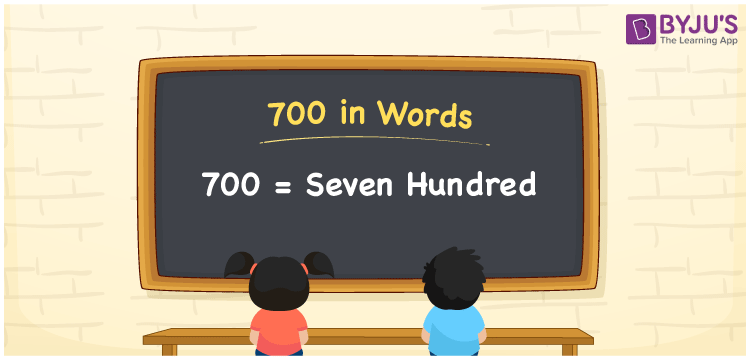# 700 in Words

700 in words is written as “Seven hundred”. In Mathematics, 700 is a cardinal number that expresses a quantity or a value or sometimes a currency. If the price of a T-shirt is Rs.700, then it can be represented as Rupees Seven hundred, in English. Learn more about Numbers In Words at BYJU’S.

 700 in Words Seven hundred Seven hundred in Numbers 700

## 700 in English Words

700 in English Words is expressed as given below:## How to Write 700 in Words?

The number 700 in words can be written using a place value system, where we can identify the position of each digit in the number. Since 700 is a three-digit number, thus,

 Hundreds Tens Ones 7 0 0

From the above table,

• 7 is at hundreds’ place
• 0 is at tens’ place
• 0 is at ones’ place

Hence, 7 comes at a hundred’s place so together we can write the number as Seven hundred.

### Expanded Form of 700

We can write the expanded form as:

7 × Hundred + 0 × Ten + 0 × One

= 7 × 100 + 0 × 10 + 0 × 1

= 700

= Seven hundred

700 is a whole number that is succeeded by 699 and preceded by 701. Learn more about the number 700 below:

• 700 in Words – Seven hundred
• Is 700 an odd number? – No
• Is 700 an even number? – Yes
• Is 700 a perfect square number? – No
• Is 700 a perfect cube number? – No
• Is 700 a prime number? – No
• Is 700 a composite number? – Yes

## Frequently Asked Questions on 700 in words

### What is 700 in words?

700 in words is given by Seven hundred.

### What is the rule to write 700 in words?

700 in words is written based on the place values of each digit. In number, 7 is at hundreds place, 0 at tens place and 0 at ones place. So, the number is read as Seven hundred in words.

### What is the value of 700 + 300 in words?

700 + 300 = 1000, i.e., One thousand